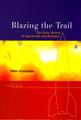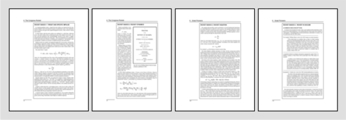# astronauticsnow.com

astronauticsnow.com/rocketequation/

Rocket equationBased on Mike Gruntman's

Blazing the Trail. The Early History of Spacecraft and Rocketry, AIAA, Reston, Va., 2004

Chapter 9, page 106

(Winner of a 2006 Award from the International Academy of Astronautics)

 Tweet

Rocket Equation

Rocket Basics 1, 2, 3, 4 (pdf)The equation that describes the rocket velocity is of such fundamental importance for rocket flight that it is called the rocket equation. It is also a contentious subject of bragging rights who was the first to derive the equation.

The rocket equation combines dynamics of a body with the varying mass and the relation between the accelerating force (thrust) and the propellant exhaust velocity. In 1813, William Moore described the relevant dynamics for constant thrust and constant propellant consumption rate acting on a rocket with the varying mass. Moore however did not relate thrust and the exhaust velocity and, therefore, did not relate the rocket velocity increment and the exhaust velocity of the propellant flow.

Derivation of the rocket equation is rather elementary. By the middle of the 19th century, problems related to rocket flight (requiring derivation of the rocket equation) had been given to university students as a standard exercise in particle dynamics (e.g., Tait and Steele 1856).

Many researchers would independently obtain this simple equation again and again throughout many years. Konstantin Tsiolkovsky described it in 1903. Robert Esnault-Pelterie, Robert Goddard, Herman Oberth, and many others independently derived the equation later.

The quantity of consumed propellant is conveniently expressed through the dimensionless mass ratio

R = M0/MB,

where M0 is the initial rocket mass; MB = M0 - MP is the rocket mass at burnout; and MP is the mass of the consumed propellant. According to the rocket equation, the rocket velocity increment delta-V would be

delta-V = Ueq ln(R)

for constant Ueq in the absence of gravity and air drag.